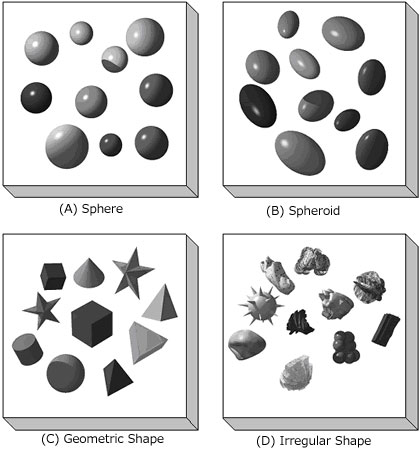# Particle Shape Problems

I often come across the question "About how much error in particle size distribution is caused by the influence of particle shape?" People, apparently, are more likely to ask this question when the measurement target (sample) is quite different from a spherical shape, for example, when it is acicular.

Behind this question lies the need or wish to measure particle size distribution with particle shape factored in because measurement of particle size distribution based on sphere-equivalent diameter is inadequate.

With the laser diffraction/scattering method, measurement of particle size distribution including shape information may be possible in the future on the assumption that comparatively simple shapes, such as spheroids that can be defined merely by their major and minor diameters, will be measured. However, it will be impossible to support the measurement of numerous and diverse particle shapes that in reality exist since multiple and, moreover, a large number of parameters will be required.

Even if it were possible to accurately measure the shape and size of particles one at a time using a principle completely different from the laser diffraction/scattering method, there would still be the problem of how to handle the shape and size of particles that are expressed by many parameters.

Alternatively, if the shape of particles was simplified, there would still be the dissatisfaction that the real shape of the parts has not been expressed. As a result, this might be almost the same as the case where the normalized particle amount is used.

If all particles were different as a result of strict measurement, then the concept of "distribution" could no longer be used. In other words, just like a case of particle size (sphere-equivalent diameter) where the measurable particle size range is divided into appropriate sections and the distribution of the normalized particle amount contained in these sections is used, particle shapes also must be divided (classified) into appropriate sections (categories).

Though this may be theoretically possible, is it practical?

Even if the shape of real particles can be divided (classified) by simple shape, such as round, triangular or square, their range of application is extremely minute.

Also, it is less practical to express the particle shape in too complicated a manner. Current particle size distribution can be expressed as a 2-dimension graph with the particle size and the normalized particle amount as the horizontal and vertical axes, respectively. It can also be expressed as a 3-dimension graph when major and minor diameters are used. Yet, it would be impossible to express particle size distribution in the form of a graph if you attempted to express particle shapes using more parameters. Even if it could be expressed as a complex mathematical table, this would merely be an arrangement of figures and cannot be said practical if people cannot grasp the meaning of its content.

In the long run, in measurement of particle size distribution where many particles (i.e. a particle group) are statistically processed and ascertained in a sense, it might be fundamentally unrealistic to handle the particle shape.

Though this is of great interest as research, I sort of feel that it will not be practical.Fig. 2 Particle Shapes